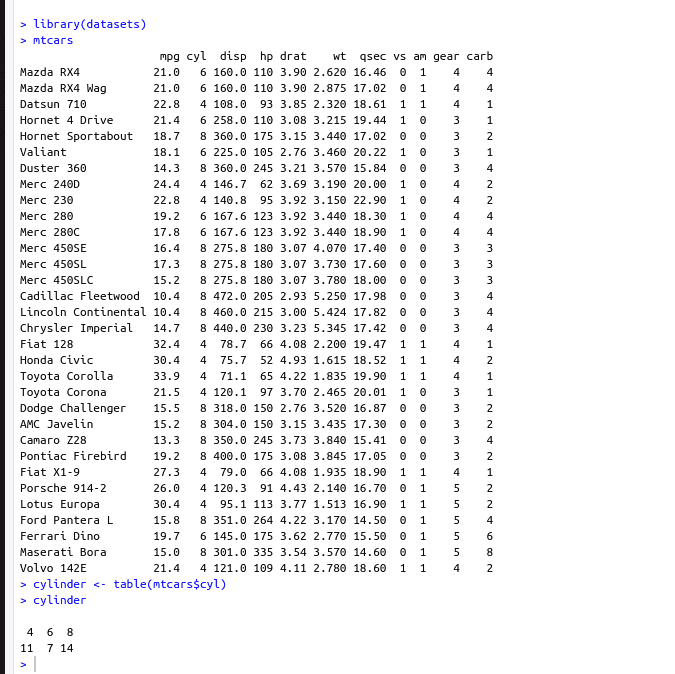# How to Use the table() Function in R

The table() function in R creates a contingency table of the counts of occurrences of values in a categorical dataset. It counts the frequency of values in a single vector or creates a cross-tabulation of counts for two or more factors. The resulting table object can be used for further analysis or visualization.

### Syntax

`` table(obj)``

### Parameters

The obj is the object to be converted to tabular form.

### Diagram1. The table in R can also represent tabular data based on conditions.
2. The table in R can also be used for cross-tabulations.
3. A frequency table with proportions can be created in R using a prop.table().
4. Data with a table in R can also be represented in three-dimensional form.

## Examples of the table in R

### Example 1

The following example gathers the data for cylinders from the mtcars dataset (32 rows) and presents it in terms of frequency using a table in R

We observe 32 observations recorded for the column cyl in the mtcars dataset, and we intend to present the frequency of these observations in a tabular form.

In the final output table, we know that there are  11 observations of cars with a 4-cylinder engine, 7 observations of cars with a 6-cylinder engine, and 14 observations of cars with an 8-cylinder engine.

``````library(datasets)
mtcars
cylinder <- table(mtcars\$cyl)
cylinder``````

Output### Example 2

Consider the situation where an event manager has to have a reference for the gender of their guests. To create a data frame in R, use the data.frame() function.

``````guestList <- data.frame(
"Name" = c("Sam", "Julie", "Rob"),
"Gender" = c("Male", "Female", "Male")
)
guestListTable <- table(guestList)
guestListTable``````

Output

``````Gender

Name  Female  Male
Julie   1     0
Rob     0     1
Sam     0     1``````

### Example 3

In the previous example, the data representation can be further extended to identify which guests on the guest list are vegan so that the event management team can cater to their dining needs accordingly. This data can be represented with the help of the table shown below.

Here, observe that R splits this data based on the values of the Vegan field and represents this data in a three-dimensional form.

``````guestList <- data.frame(
"Name" = c("Sam", "Julie", "Rob"),
"Gender" = c("Male", "Female", "Male"),
"Vegan" = c("Yes", "Yes", "No")
)

guestListTable <- table(guestList)
guestListTable``````

OutputThat’s it for this tutorial.